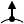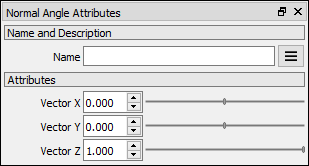# Normal Angle node

Left Toolbar >Normal Nodes >Normal Angle

The Normal Angle node computes the angle of the normal and an user defined vector and returns a VALUE image with the angles in radians.

inputimage

outputimage

## Procedure

### To calculate the normal angle:

1. On the left toolbar choose Normal Nodes > Normal Curvature.>2. Drag the Normal Angle icon into the Node Graph.3. Connect a Normal image to the input socket in the Normal Angle node.

4. Connect the Output.

image
An NORMAL image.

## Outputs

image
A VALUE image with the angle in radians.

## Attributes PanelVector X
The vector's x component.

Vector Y
The vector's y component.

Vector Z
The vector's z component.

## Error Messages

The Normal Angle node logs an error message if the input image is not a NORMAL image.